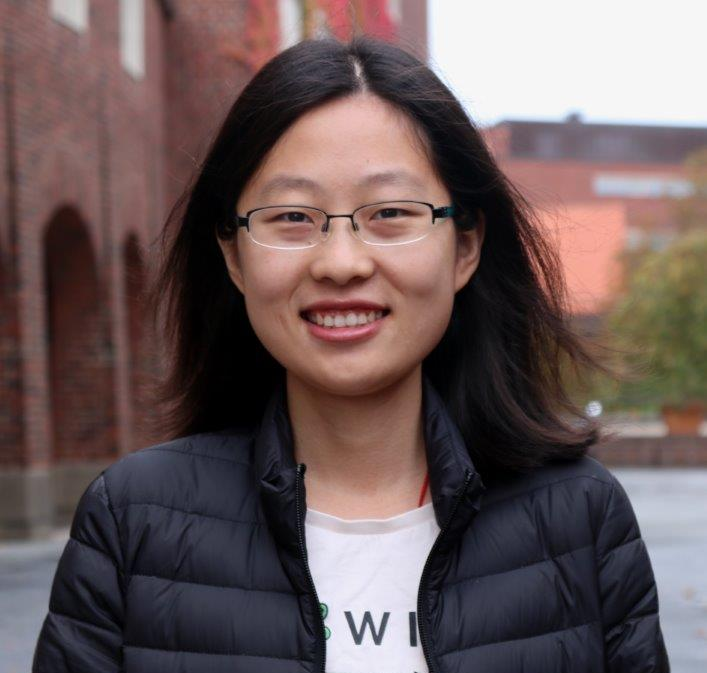# Yuanyuan Xu 许媛媛

### Contact Info:

Institute of Science and Technology Austria,
Am Campus 1,
3400 Klosterneuburg, Austria
Office: I21.O3.114
Email: yuanyuan.xu AT ist DOT ac DOT at

I am currently a postdoc in the group of László Erdős at Institute of Science and Technology Austria (IST Austria).

Before that, I was a postdoc at KTH Royal Institute of Technology, working with Kevin Schnelli.
I obtained my Ph.D. degree in applied mathematics from University of California, Davis in 2018, under the supervision of Alexander Soshnikov.
I received my B.S. degree in applied mathematics from University of Science and Technology of China (USTC) in 2013.

I am interested in probability theory, high-dimensional statistics, and mathematical physics, with a focus on random matrix theory.

### Publications and preprints:

1. Convergence rate to the Tracy-Widom laws for the largest eigenvalue of sample covariance matrices,
with Kevin Schnelli,
accepted by Annals of Applied Probability (2022).

2. Small deviation estimates for the largest eigenvalue of Wigner matrices,
with László Erdős,
accepted by Bernoulli (2022).

3. Convergence rate to the Tracy-Widom laws for the largest eigenvalue of Wigner matrices,
with Kevin Schnelli,
accepted by Communications in Mathematical Physics (2022).

4. Central limit theorem for mesoscopic eigenvalue statistics of free sum of matrices,
with Zhigang Bao and Kevin Schnelli,
International Mathematics Research Notices, Vol. 2022, No. 7, 5320-5382 (2022).

5. On fluctuations of global and mesoscopic linear statistics of generalized Wigner matrices,
with Yiting Li,
Bernoulli, Vol. 27, No. 2, 1057-1076 (2021).

6. Central limit theorem for mesoscopic eigenvalue statistics of deformed Wigner matrices and sample covariance matrices,
with Yiting Li and Kevin Schnelli,
Annales de l’Institut Henri Poincaré - Probabilités et Statistiques, Vol. 57, No. 1, 506–546 (2021).

7. Gaussian Approximation of the Distribution of Strongly Repelling Particles on the Unit Circle,
with Alexander Soshnikov,
Theory of Probability and Its Applications, Vol. 65, No. 4, 588-615 (2021).

• None.

### Previous teaching:

• MAT 21B Calculus: Integral Calculus, Summer Session I, UC Davis, 2016.

• Teaching assistant at UC Davis (2013-2017):
• MAT 17C Calculus for Biology and Medicine
• MAT 21A Calculus: Differential Calculus
• MAT 21B Calculus: Integral Calculus
• MAT 22A Linear Algebra
• MAT 22B Differential Equations
• MAT 125B Real Analysis
• MAT 207A Applied Mathematics

Last updated : April 8, 2022.